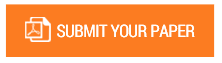A Mapping Scheme for Mapping from Subsets of Complex Matrix Spaces Characterized by a Given set of Global Mass and Alignment Factors to the set of Hermitian, Positive Definite and Positive Semi Definite, Unit Trace, Complex Matrices of order 2

Authors : Debopam Ghosh

Volume/Issue : Volume 6 - 2021, Issue 1 - January

Scribd : https://bit.ly/39eKtqV

The present research article discuss a mapping scheme from the subsets of the complex Matrix space M C m n characterized by set of Global Mass factor and Global Alignment factor, denoted as M r c , to the subset of the Matrix space 2 2 M C which consist of all Hermitian, unit trace, positive definite and positive semi definite matrices of order 2, denoted as 2 2 ˆ S M C . The Mathematical formalism is presented and illustrated through appropriate Numerical examples

Keywords : Global Mass Factor of a Matrix, Global Alignment Factor of a Matrix, Effective Global Mass Factor of a Matrix, Hermitian Matrices, Positive Definite Matrices, Positive Semi definite Matrices.

#### CALL FOR PAPERS

Paper Submission Last Date
31 - May - 2022